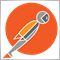# MTF fix using GlobalVariableGet470

Hi,

Can somebody please tell me if my coding solution is correct in solving a 4 H MTF call for an EA that runs on a 1 H chart, or at least clarify further the following:

I run a separate EA to call the 4 H CCI to my 1 H based EA as shown below:

```double CCI_H4=0;
if (iBars(NULL,PERIOD_H4)>14) CCI_H4 = iCCI(NULL,PERIOD_H4,14,PRICE_MEDIAN,1);
GlobalVariableSet("CCI_H4",CCI_H4);```

Then I run the following code in my EA:

```void CheckForSignal()
{
double Cci=GlobalVariableGet("CCI_H4");
static datetime candletime=0;
if(candletime!=Time && Cci!=0)
{
//---4 H Oscilator
//double Cci=iCCI(NULL,PERIOD_H4,14,PRICE_MEDIAN,1);
//---1 Hour Trend
//--- Ema 200
double Ema1hr_200=iMA(NULL,PERIOD_H1,200,0,MODE_EMA,PRICE_MEDIAN,1);
//---Price
double Price=(High+Low)/2;
double PriceBack=(High+Low)/2;
//---Fibionacci MAs
double Fib5H1=iMA(NULL,PERIOD_H1,5,0,MODE_EMA,PRICE_MEDIAN,1);
double Fib8H1=iMA(NULL,PERIOD_H1,8,0,MODE_EMA,PRICE_MEDIAN,1);
double Fib13H1=iMA(NULL,PERIOD_H1,13,0,MODE_EMA,PRICE_MEDIAN,1);
//---Trigger Confirmation
//---Sar
double SarH1=iSAR(NULL,PERIOD_H1,0.07,0.2,1);
double SarBackH1=iSAR(NULL,PERIOD_H1,0.07,0.2,2);

//Did it make an up arrow on candle 1?

if(Price>Ema1hr_200)
if(Cci<100)
if(Fib5H1>Fib8H1 && Fib8H1>Fib13H1)
if(SarBackH1>SarH1 && SarH1<Price)
//---

The question to clarify is that is this a workable solution? in that I read in MQL4 reference the following:

Note

Global variables exist in the client terminal during 4 weeks since their last use, then they are automatically deleted.

Does this mean that they are deleted every 4 weeks or if they are used within a 4 week period then they are not deleted?

Any advice would be very much appreciated, I look forward to any help on this topic.

Regards Mike20208

1. Global variables are for interprocess communication. Why do you need to get the value and save it for other code?  What part of "since their last use," is unclear?

2. On MT4: Unless the current chart is that specific pair/TF referenced, you must handle 4066/4073 errors.470

whroeder1:
1. Global variables are for interprocess communication. Why do you need to get the value and save it for other code?  What part of "since their last use," is unclear?

2. On MT4: Unless the current chart is that specific pair/TF referenced, you must handle 4066/4073 errors.

Why do you need to get the value and save it for other code?

This is a simpler working solution.......correct? or is there some other unforeseen reason that I can not use this code to fix a MTF issue?2392

Michael Green:

Why do you need to get the value and save it for other code?

This is a simpler working solution.......correct? or is there some other unforeseen reason that I can not use this code to fix a MTF issue?

The better working solution is to simply get the 4 hour cci data from the same EA instead of relying on an external program.470

ok so how would I code this?2392

Michael Green:
ok so how would I code this?